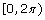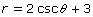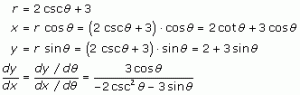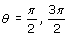# Problem 13: Polar Derivatives

2011-2012 Find all angles on the interval  at which the tangent line to the graph of the polar equation  is horizontal. Solution: Express the polar equations parametrically (in terms ofx and y) and calculate the slope of the polar equation. The tangent lines to the polar graph are horizontal when the numerator of this derivative is … Continue reading "Problem 13: Polar Derivatives"##2011-2012

Find all angles on the intervalat which the tangent line to the graph of the polar equationis horizontal.

### Solution:

Express the polar equations parametrically (in terms ofx and y) and calculate the slope of the polar equation.The tangent lines to the polar graph are horizontal when the numerator of this derivative is equal to 0. In other words, at.

The video may take a few seconds to load.Having trouble Viewing Video content? Some browsers do not support this version – Try a different browser.